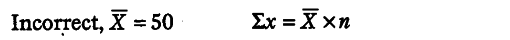# The mean of 200 items was 50.Later it was discovered that two items,

The mean of 200 items was 50. Later it was discovered that two items were misread as 92 and 8 instead of 192 and 88. Find the correct mean.Σx = 200 x 50 = 10000

Correct Mean = ΣX - Sum of Incorrect Values + Sum of Correct Value / N
= 10000 - (92 + 8 ) + (192+88)/200 = 10000-100+280/200 = 10180/200

Correct Mean = 50.9